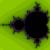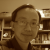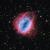# Cube to Rectangular PrismThis cube can be used in a classroom to demonstrate to students the differences between cubes and rectangular prisms.

The first part of the program makes a solid cube. The second part of the program was repeated with the line function (rather than the face function) to draw a copy of the cube next to the original cube.

Through the use of this virtual world, students are able to discuss the properties of a cube and also the properties of a rectangular prism.

Students can conclude that:

Cube

• Has 6 equal faces of the same length
• Has 8 verticies
• Has 12 edges

Rectangular Prism

• Has 6 faces, only some of the sides are the same length
• Has 8 verticies
• Has 12 edges

This program provides the students an opportunity to compare and discuss the relationships between a cube and rectangular prism. Students can also have discussions about how to make a rectangular prism and the difference between a solid shape and a net..

• Students can create physical rectangular prisms using unifix cubes to check their original assumptions of a rectangular prism.
• Students can attempt drawing the net of a rectangular prism.
• Students can use the information about the relationship between a cube and a rectangular prism to the relationship between a square and a rectangle.### Is a cube also a rectangular prism?

Thanks for sharing the teaching ideas.I think if students can contruct this virtual world, they will get better spatial sense from the 3D movements used. Because the distance involved in all movements is 1 meter and the angle involved is 90 degrees, so I think students would have learnt of the length of edge and angle of the rectangular prism.

BTW,  is a cube also a rectangular prism? anyone?YES!